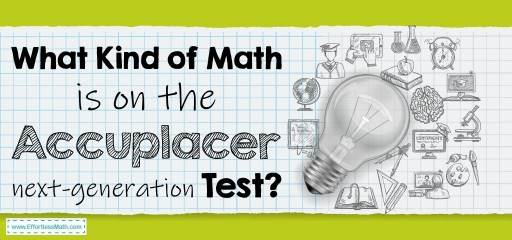# What Kind of Math Is on the Accuplacer Next-Generation Test?The next-generation Accuplacer test is a standardized placement test that helps determine the place of students at the university level. This test determines the exact level of students in the classes that suits their skills. The next-generation Accuplacer is a type of computer-adaptive test and the test takers’ answer to each question determines the level of difficulty of the next question. This test tries to determine exactly where your skill level is by changing the difficulty of each question based on your answers. The main test of ACCUPLACER has 10 sub-tests. All of these sub-tests include multiple-choice questions, except WritePlacer which is a writing test. There is no time limit for answering most of these tests.

There are five main sub-sections in the Accuplacer test: Arithmetic, Quantitative Reasoning, Algebra, And Statistics (QAS), Advanced Algebra and Functions, Reading, Writing.

As you can see, the math-related subsections of the test include:

• Arithmetic
• Quantitative Reasoning, Algebra, And Statistics (QAS)

In the math section of the test, test takers are not allowed to use personal calculators, but the test center provides an on-screen calculator for students for some Questions.

## Arithmetic Subtest

This section assesses your ability to do arithmetic computations and problem-solving. The purpose of including this section in the test is whether or not you are proficient in performing simple calculations manually. The questions in this part of the test include topics such as decimal operations, Fraction operations, Number Comparisons and Equivalents, Percent, Whole Number Operations.

## Quantitative Reasoning, Algebra and Statistics (QAS) Subtest

The purpose of this subtest is to determine your level of proficiency in calculating questions related to rational numbers and exponents. To succeed in this field, you must be able to solve algebraic expressions and graph linear equations. You should also be familiar with geometry problems, sets of probabilities, and descriptive statistics.

## Advanced Algebra and Functions Subtest

In this section, you are mostly dealing with linear equations and their applications. Factoring and solving quadratic, radical, rational, and polynomial equations is also very important in this section. Trigonometry content is also included in this section.

## FAQs:

### Is the Accuplacer next-generation Math test hard?

It can be challenging, but if you study the lessons of this test well, you can pass this test successfully.

### How do I study for the Accuplacer next-generation Math?

How you prepare for the Accuplacer math test is very important. You can use good prep books to prepare well for the Accuplacer Math test. You can also use online practice tests for further practice after studying the Accuplacer exam courses.

### How is the Accuplacer’s next-generation Test Scored?

The score range for writing, reading, quantitative reasoning, arithmetic, algebra and statistics, advanced algebra, and functions is 200-300. for more information, you can check here.

## The Best Books to Ace the Accuplacer Math Test

### What people say about "What Kind of Math Is on the Accuplacer Next-Generation Test? - Effortless Math: We Help Students Learn to LOVE Mathematics"?

No one replied yet.

X
52% OFF

Limited time only!

Save Over 52%

SAVE \$40

It was \$76.99 now it is \$36.99